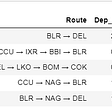# Polynomial terms in a regression model

Let’s say you have a dataset containing test scores of students and number of hours they studied a day before the test.

Here is how the relationship between them looks like.

Test scores improve till a certain number of hours after which more hours start to have a detrimental effect on student’s performance in the test.

If you model this relationship using a linear regression algorithm, this is how it will typically look like.

Here is the model summary.

or, score = 66.9915 + 1.6888*hours_studied

The adjusted R-square for this model is 0.1 which means that the model captures this relationship very poorly. This is not surprising, given the non-linear nature of the relationship.

Does that mean you cannot improve this model within the contours of a linear regression algorithm? Not really.

You can be creative with your features, transform them and add them to your model. Here, since we know the relationship is non-linear and scores start dropping beyond a certain number of hours we try adding a square term (i.e. square of hours studied) to the model.

The model summary looks like this.

or, score = 42.0983 + 16.3319*hours_studied — 1.4498*hours_studied²

The model has two features now -

1. Hours studied in its original form
2. Square of the hours studied

Notice the significant improvement in adjusted R-square from 0.1 to 0.635.

It is intuitive from the coefficients that the score increases as hours studied increase initially because of the positive coefficient on hours studied. However, at higher values of hours studied the square term due to its negative coefficient starts dragging down the overall score.

This model captures the relationship much more accurately than the earlier one.

Visually this is how things look like now.

Note : We did not use a new algorithm to fit this model, it is still a linear regression model based on ordinary least squares. All we did is added a transformed feature (a square term) and fit the model.

Here is a side by side comparison of the two models.

Which one of these would you prefer to predict test scores? I am pretty sure the latter.

--

--

--

## More from Analytics Vidhya

Analytics Vidhya is a community of Analytics and Data Science professionals. We are building the next-gen data science ecosystem https://www.analyticsvidhya.com

## What should a Resume look like for an Entry Level Data Scientist Position?## dClimate FAQ’s## Detailed Explanation of Random Forests Features importance Bias## Week in OSINT #2020–25## Data Manipulation With Python Pandas## Exploring IPL through Exploratory Data Analysis## Survey of learning from only a few examples with computer vision applicationsLifelong learner

## Binary Classification Through Logistic Regression — Analytics Mag## Why Implementing Random Forest Over Decision Tree?## Gini Gain vs Gini Impurity | Decision Tree — A Simple Explanation## Prediction of Airline Fare-Achieved 98% R2 score through applying Feature Engineering concepts